# OpenCV4学习笔记（71）——散焦图像去模糊滤波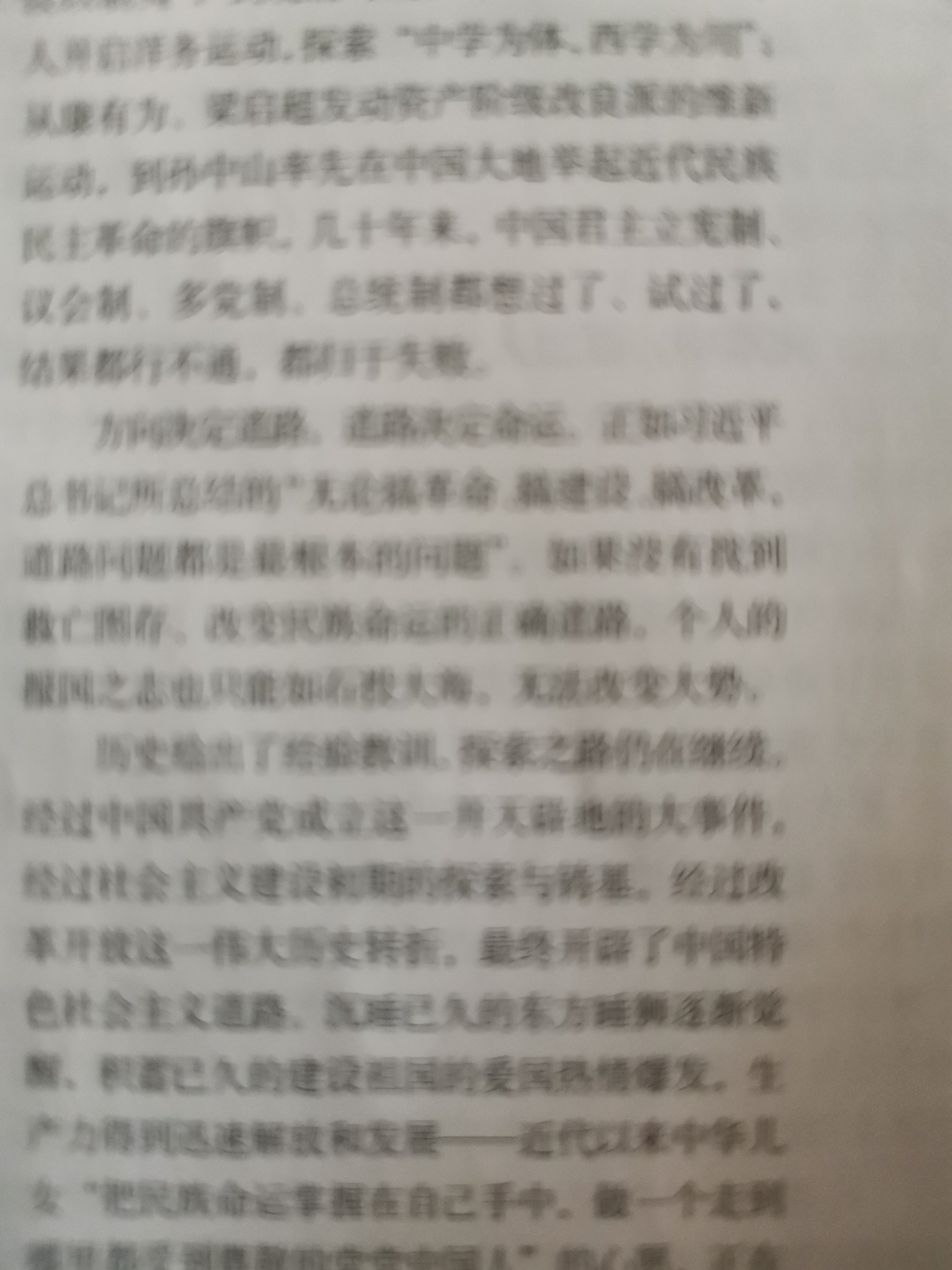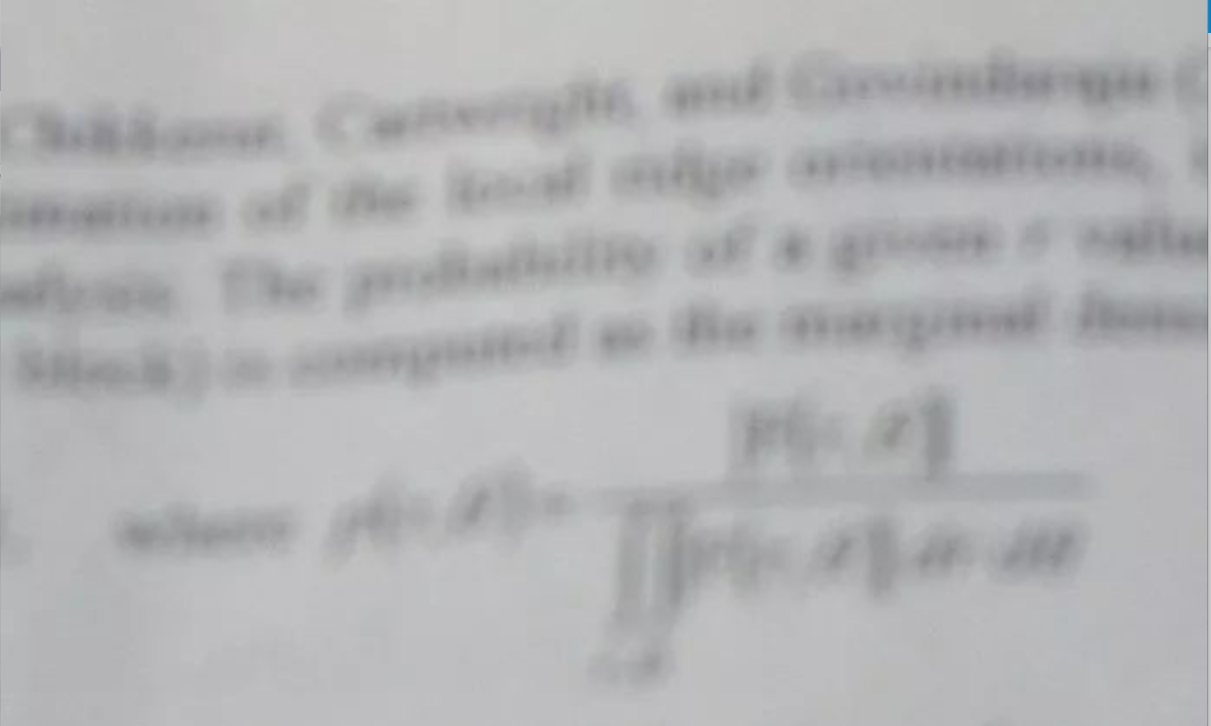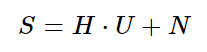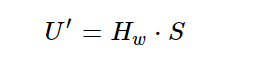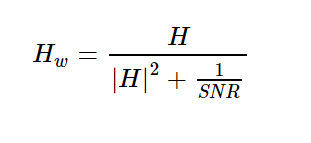``````void calcPSF(Mat& outputImg, Size filterSize, int R)
{
Mat h(filterSize, CV_32F, Scalar(0));
Point point(filterSize.width / 2, filterSize.height / 2);
circle(h, point, R, 255, -1, 8);
Scalar summa = sum(h);
outputImg = h / summa;
}
``````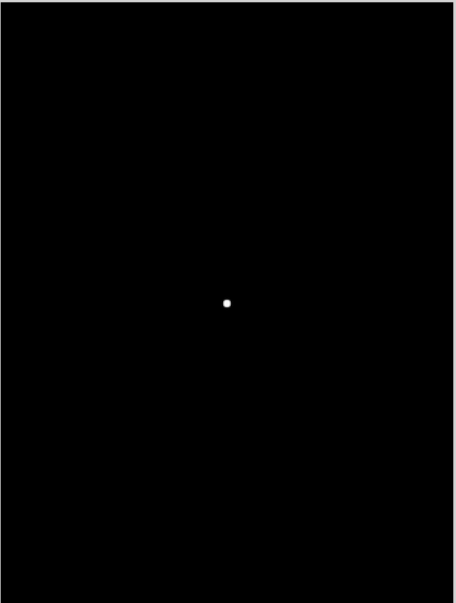``````void calcWnrFilter(const Mat& input_h_PSF, Mat& output_G, double snr)
{
Mat h_PSF_shifted;
fftshift(input_h_PSF, h_PSF_shifted);
Mat planes = { Mat_<float>(h_PSF_shifted.clone()), Mat::zeros(h_PSF_shifted.size(), CV_32F) };
Mat complexI;
merge(planes, 2, complexI);
dft(complexI, complexI);
split(complexI, planes);
Mat denom;
pow(abs(planes), 2, denom);
denom += 1.0 / double(snr);
divide(planes, denom, output_G);
}
``````

``````void fftshift(const Mat& inputImg, Mat& outputImg)
{
outputImg = inputImg.clone();
int cx = outputImg.cols / 2;
int cy = outputImg.rows / 2;
Mat q0(outputImg, Rect(0, 0, cx, cy));
Mat q1(outputImg, Rect(cx, 0, cx, cy));
Mat q2(outputImg, Rect(0, cy, cx, cy));
Mat q3(outputImg, Rect(cx, cy, cx, cy));
Mat tmp;
q0.copyTo(tmp);
q3.copyTo(q0);
tmp.copyTo(q3);
q1.copyTo(tmp);
q2.copyTo(q1);
tmp.copyTo(q2);
}
````````````void filter2DFreq(const Mat& inputImg, Mat& outputImg, const Mat& H)
{
Mat planes = { Mat_<float>(inputImg.clone()), Mat::zeros(inputImg.size(), CV_32F) };
Mat complexI;
merge(planes, 2, complexI);
dft(complexI, complexI, DFT_SCALE);
Mat planesH = { Mat_<float>(H.clone()), Mat::zeros(H.size(), CV_32F) };
Mat complexH;
merge(planesH, 2, complexH);
Mat complexIH;
mulSpectrums(complexI, complexH, complexIH, 0);
idft(complexIH, complexIH);
split(complexIH, planes);
outputImg = planes;
}
``````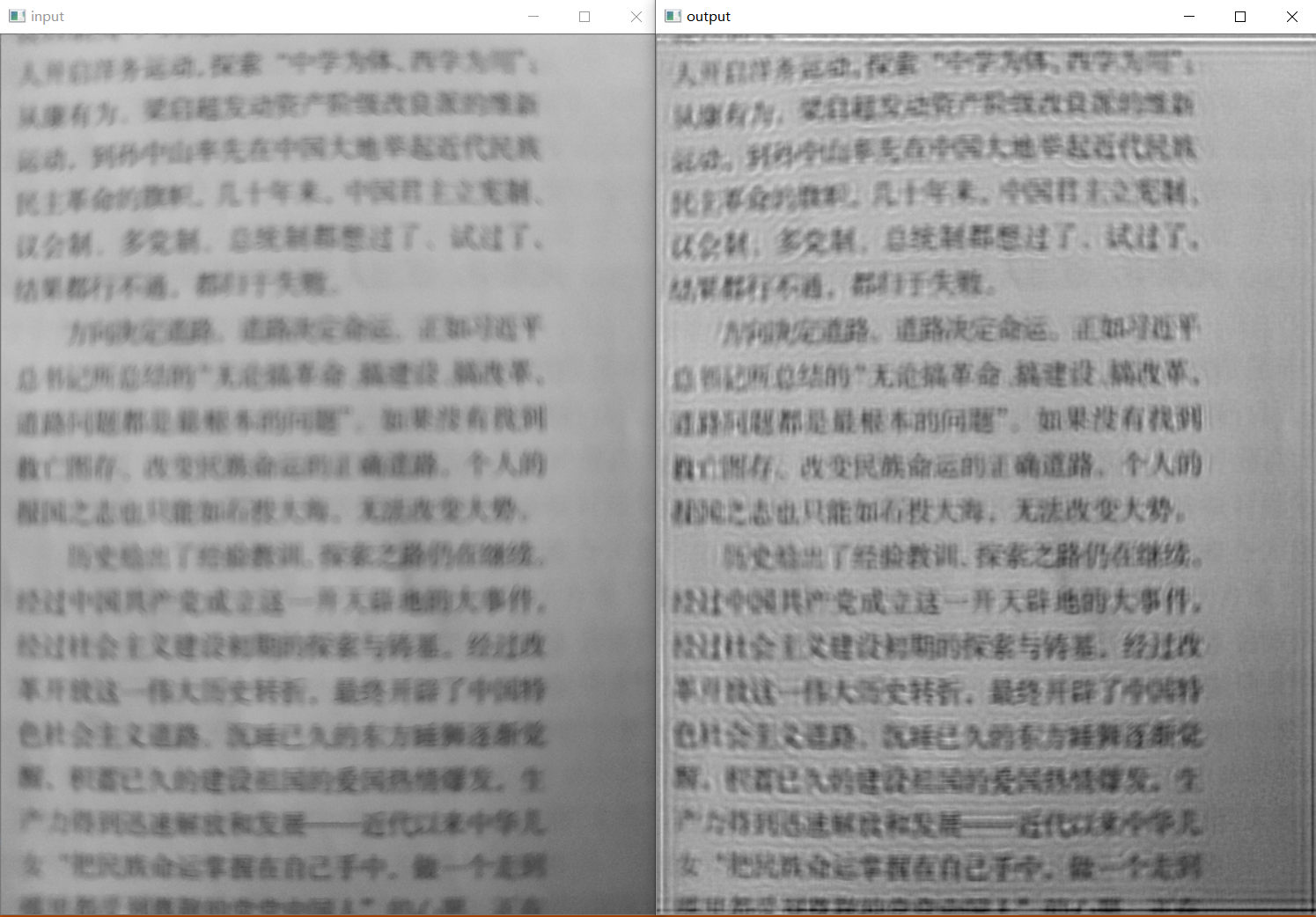``````void edgetaper(const Mat& inputImg, Mat& outputImg, double gamma, double beta)
{
int Nx = inputImg.cols;
int Ny = inputImg.rows;
Mat w1(1, Nx, CV_32F, Scalar(0));
Mat w2(Ny, 1, CV_32F, Scalar(0));
float* p1 = w1.ptr<float>(0);
float* p2 = w2.ptr<float>(0);
float dx = float(2.0 * CV_PI / Nx);
float x = float(-CV_PI);
for (int i = 0; i < Nx; i++)
{
p1[i] = float(0.5 * (tanh((x + gamma / 2) / beta) - tanh((x - gamma / 2) / beta)));
x += dx;
}
float dy = float(2.0 * CV_PI / Ny);
float y = float(-CV_PI);
for (int i = 0; i < Ny; i++)
{
p2[i] = float(0.5 * (tanh((y + gamma / 2) / beta) - tanh((y - gamma / 2) / beta)));
y += dy;
}
Mat w = w2 * w1;
multiply(inputImg, w, outputImg);
}
``````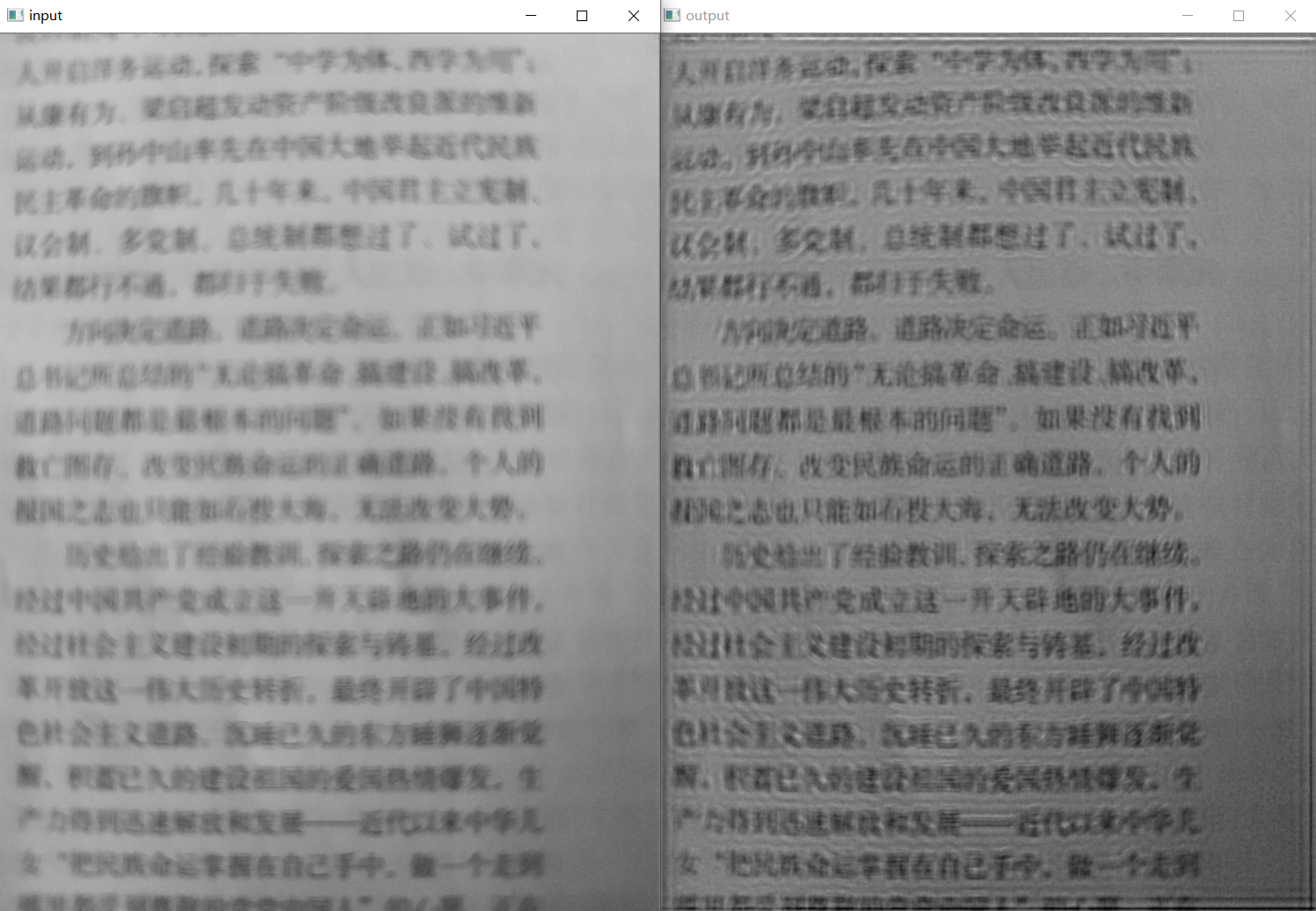``````#include<opencv.hpp>
#include<stdlib.h>
#include<string>
#include<vector>
using namespace std;
using namespace cv;

void calcPSF(Mat& outputImg, Size filterSize, int R);
void fftshift(const Mat& inputImg, Mat& outputImg);
void filter2DFreq(const Mat& inputImg, Mat& outputImg, const Mat& H);
void calcWnrFilter(const Mat& input_h_PSF, Mat& output_G, double nsr);
void edgetaper(const Mat& inputImg, Mat& outputImg, double gamma = 5.0, double beta = 5.2);
int main()
{
resize(imgIn, imgIn, Size(600, 800));
Mat imgOut;
//截取处理区域，对宽高取偶数
Rect roi = Rect(0, 0, imgIn.cols & -2, imgIn.rows & -2);
Mat Hw, h;
int R = 5;                                 //圆形点扩散函数PSF的半径
int SNR =35;             //圆形点扩散函数PSF的信噪比

//创建圆形点扩散函数PSF
calcPSF(h, roi.size(), R);
//创建维纳滤波器
calcWnrFilter(h, Hw, SNR);
//削弱振铃效应
edgetaper(Hw, Hw);
//进行滤波
filter2DFreq(imgIn(roi), imgOut, Hw);
//转换类型并归一化
imgOut.convertTo(imgOut, CV_8UC1);
normalize(imgOut, imgOut, 0, 255, NORM_MINMAX);

//namedWindow("input", WINDOW_FREERATIO);
imshow("input", imgIn);
//namedWindow("output", WINDOW_FREERATIO);
imshow("output", imgOut);

waitKey(0);
return 0;
}

//对于散焦图像去模糊时使用点扩散函数，如果针对运动图像去模糊可以使用带有角度和长度的点扩散函数，角度即是物体运动方向、长度取决于运动速度
void calcPSF(Mat& outputImg, Size filterSize, int R)
{
Mat h(filterSize, CV_32F, Scalar(0));
Point point(filterSize.width / 2, filterSize.height / 2);
circle(h, point, R, 255, -1, 8);
Scalar summa = sum(h);
outputImg = h / summa;
}

void calcWnrFilter(const Mat& input_h_PSF, Mat& output_G, double snr)
{
Mat h_PSF_shifted;
fftshift(input_h_PSF, h_PSF_shifted);
Mat planes = { Mat_<float>(h_PSF_shifted.clone()), Mat::zeros(h_PSF_shifted.size(), CV_32F) };
Mat complexI;
merge(planes, 2, complexI);
dft(complexI, complexI);
split(complexI, planes);
Mat denom;
pow(abs(planes), 2, denom);
denom += 1.0 / double(snr);
divide(planes, denom, output_G);
}

//重新排列傅立叶图像的象限，使原点位于图像中心
void fftshift(const Mat& inputImg, Mat& outputImg)
{
outputImg = inputImg.clone();
int cx = outputImg.cols / 2;
int cy = outputImg.rows / 2;
Mat q0(outputImg, Rect(0, 0, cx, cy));
Mat q1(outputImg, Rect(cx, 0, cx, cy));
Mat q2(outputImg, Rect(0, cy, cx, cy));
Mat q3(outputImg, Rect(cx, cy, cx, cy));
Mat tmp;
q0.copyTo(tmp);
q3.copyTo(q0);
tmp.copyTo(q3);
q1.copyTo(tmp);
q2.copyTo(q1);
tmp.copyTo(q2);
}

void filter2DFreq(const Mat& inputImg, Mat& outputImg, const Mat& H)
{
Mat planes = { Mat_<float>(inputImg.clone()), Mat::zeros(inputImg.size(), CV_32F) };
Mat complexI;
merge(planes, 2, complexI);
dft(complexI, complexI, DFT_SCALE);
Mat planesH = { Mat_<float>(H.clone()), Mat::zeros(H.size(), CV_32F) };
Mat complexH;
merge(planesH, 2, complexH);
Mat complexIH;
mulSpectrums(complexI, complexH, complexIH, 0);
idft(complexIH, complexIH);
split(complexIH, planes);
outputImg = planes;
}

//使恢复滤波器的边缘逐渐变细，以减少还原图像中的振铃效果
void edgetaper(const Mat& inputImg, Mat& outputImg, double gamma, double beta)
{
int Nx = inputImg.cols;
int Ny = inputImg.rows;
Mat w1(1, Nx, CV_32F, Scalar(0));
Mat w2(Ny, 1, CV_32F, Scalar(0));
float* p1 = w1.ptr<float>(0);
float* p2 = w2.ptr<float>(0);
float dx = float(2.0 * CV_PI / Nx);
float x = float(-CV_PI);
for (int i = 0; i < Nx; i++)
{
p1[i] = float(0.5 * (tanh((x + gamma / 2) / beta) - tanh((x - gamma / 2) / beta)));
x += dx;
}
float dy = float(2.0 * CV_PI / Ny);
float y = float(-CV_PI);
for (int i = 0; i < Ny; i++)
{
p2[i] = float(0.5 * (tanh((y + gamma / 2) / beta) - tanh((y - gamma / 2) / beta)));
y += dy;
}
Mat w = w2 * w1;
multiply(inputImg, w, outputImg);
}
``````

（1）对于散焦图像而言，因为当它拍摄的时候就已经失去了过多的信息，导致这种图像所包含的信息是非常不完整的，也就导致如果我们想要对散焦图像进行清晰化有着非常大的困难。
（2）对于这样的模糊图像，我们可以尝试通过频域滤波在一定程度上恢复图像信息，但远远无法与未失真图像相媲美。
（3）这种通过频域滤波的方法，需要我们寻找到一个最合适的PSF点扩散函数对应的半径R和信噪比SNR，其中半径R的影响权重更大，可以优先调节R的值。这个调整参数的过程相当考验我们的耐心。
（4）对于频域滤波器的选择和处理也很重要，要尽量避免振铃效应对图像结果的影响，尽量让滤波器的过渡更加平滑。

PS：本人的注释比较杂，既有自己的心得体会也有网上查阅资料时摘抄下的知识内容，所以如有雷同，纯属我向前辈学习的致敬，如果有前辈觉得我的笔记内容侵犯了您的知识产权，请和我联系，我会将涉及到的博文内容删除，谢谢！